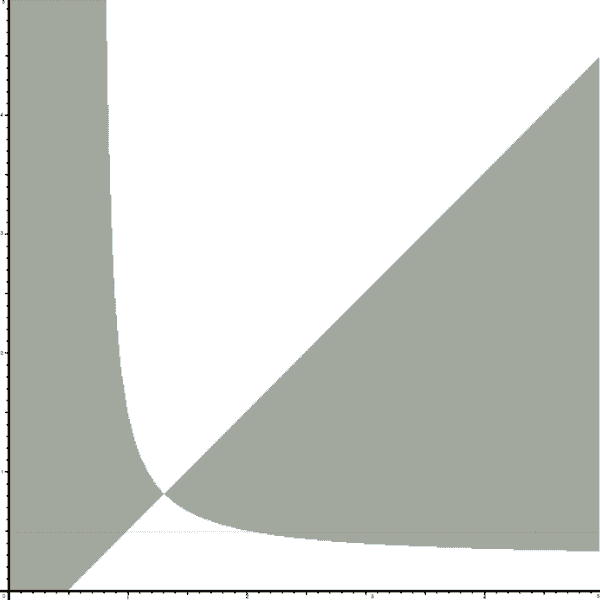# [Wolfram Mathematica] Using Newton's method to solve non-linear system

Hernaner28
Hi. This is not actually not part of the homework; but it's something I'd like to do.
I have to solve the following system using Newton-Raphson's method:

$$\begin{matrix} \frac{X}{\mu }+Y=1 \\ X=\left( \lambda -\left( K-1 \right)X \right)Y \\ \end{matrix}$$

Surfing the internet I found this precious Wolfram Demonstration:
http://demonstrations.wolfram.com/IterationsOfNewtonsMethodForTwoNonlinearEquations/

which is an interactive plot where you can select the starting point and see how and where it converges.

So I took the code and put those functions of mine:

Code:
Manipulate[
Quiet@DynamicModule[{sol},
sol = Prepend[
Reap[FindRoot[f, {vars, start}\[Transpose],
StepMonitor :> Sow[vars]]][[2, 1]], start];
Show[g1, g2,
Graphics[{PointSize[Medium], Point[sol], Thickness[Medium],
Line[sol], Style[Text[start, {-20, 20}, {-1, 1}], 12]}]]],
{{start, {3.7, 5.7}}, Locator},
SaveDefinitions -> True,
SynchronousInitialization -> False,
TrackedSymbols -> True,
Initialization :> {
f = {x + y - 1, x - y*(0.99 - 4*x) },
vars = {x, y},
root = vars /. FindRoot[f, {vars, {1, 1}}\[Transpose]],
pp = {},
Do[sol = vars /. FindRoot[f, {vars, {x0, y0}}\[Transpose]];
If[Norm[sol - root] < 10^-7, pp = {pp, {x0, y0}}], {x0, -20,
20, .12}, {y0, -20, 20, .12}],
g1 = ListPlot[Partition[Flatten[pp], 2], AspectRatio -> Automatic,
PlotStyle -> PointSize[Tiny],
PlotRange -> {{-20, 20}, {-20, 20}},
Ticks -> {Range[-20, 20], Range[-20, 20]},
ImageSize -> {480, 480}],
g2 = ContourPlot[
Evaluate[Thread[f == 0]], {x, -20, 20}, {y, -20, 20},
ContourStyle -> {{Thick, Green}, {Thick, Orange}}]}]

It uses the FindRoot command which is supposed to use Newton's method but it actually does not purely. I checked this because I wrote the method on Octave and the region of convergence is the following:As you can see it's "solid" whereas in the Wolfram Demonstration you can see stripes so FindRoot clearly does not use Newton's method.

So now I want to replace that FindRoot command to match the existing code but using the pure Newton's method. I just don't know where to start because I barely understand that code.
So if someone is a pro in Wolfram I would really appreciate him/her !!

#### Attachments

Bill Simpson
I think what you want to replace is the

Code:
FindRoot[f, {vars, start}\[Transpose], StepMonitor :> Sow[vars]]

The StepMonitor:>Sow[vars] is going to put the result of each successive iteration "into the bag" and when finished Reap[][[2,1]] around this will extract the contents of the bag for Show to then display.

So if you replace all of that FindRoot[] with your code that uses Newton and puts successive {x,y} points into the bag with Sow[vars] then you should be close to what you want.

You could start with something that just uses a For[Sow[{x,y}],{x,1,3},{y,1,4}]] instead of the FindRoot[] to put points in the bag to verify this much of the process. Then you could replace that with more of your Newton.

There are two additional FindRoot[] down further in the code. It looks like those are being used for graphics showing either the beginning or the end of the iterative process. It doesn't look like those are absolutely critical to what you are trying to do. You might try gently commenting those out of the code and see how the graphics change.

The Demonstrations project often provide something that looks cute, but all the stuff they have to shovel in to create the interactive graphical result almost always makes this very complicated and difficult to reverse engineer for even a slightly different purpose. This is very different from providing brilliantly simple examples to show how algorithms can be implemented and that you can easily see how to incorporate into what you need to do. But cute graphics are what many people seem to think they want to see.

Almost all the internal algorithms used for things like FindRoot[] are very complicated and not adequately documented for the user to be able to tell exactly how they work. Many of these supposedly take dozens or even hundreds of pages of code to implement. There isn't even documentation of the available arguments "somemethod" that can be used with Method->"somemethod" or even a rough description of what each somemethod might do, you have to guess from the name and do trial and error experimentation.